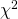##profiles

In the following table you can find the text-readable profiles that you can use to reproduce our 1D and 2D plots. All the files are normalized so that the best fit (in both normal and inverted ordering) always corresponds to . All the profiles can be downloaded from https://doi.org/10.5281/zenodo.4726908. See the page Cite this page for instructions on citing these results if you use them in a publication. 1D profiles The 1D profiles are written in the format parameter_value chi^2 parameter normal ordering inverted ordering dm21-NO.dat dm21-IO.dat dm31-NO.dat dm31-IOdat sq12-NO.dat sq12-IO.dat sq13-NO.dat sq13-IO.dat sq23-NO.dat sq23-IO.dat del-NO.dat del-IO.dat 2D profiles The 2D profiles are written in theRead More →

## Solar neutrino experiments

Here we present the result of our simulation of solar neutrino experiments and the long baseline reactor experiment KamLAND. In our analysis we include data from the following experiments: -KamLAND: Data from the analysis performed here. -Solar data: We include data from the Homestake chlorine detector (paper), from GALLEX (paper), from SAGE (paper), from Super-Kamiokande (paper1, paper2, paper3, paper4), from SNO (paper) and Borexino (paper). For most of these experiments we use the final data samples. From this list the only ones still taking data are Super-Kamiokande and Borexino. The result of our simulation is presented in the following figure. We present the result from theRead More →

## Long baseline accelerator experiments

Here we present the result of our simulation of long baseline neutrino experiments. In our analysis we include data from the following experiments: -K2K: Data from the analysis performed here. -MINOS: Data from the analysis performed here. -T2K: Data from the analysis performed here. -NOA: Data from the analysis performed here. The result of our simulation is presented in the next figure (left for normal ordering, right for inverted ordering). We do not plot the result of the K2K experiment, since it is not competitive with the rest of the experiments. Note that in the case of K2K and MINOS we include the full andRead More →

## Reactor neutrino experiments

Here we present the result of our simulation of reactor neutrino experiments. In our analysis we include data from the following experiments: -Daya Bay: Data from the analysis performed here. -RENO: Data from the analysis performed here. The result of our simulation is presented in the next figure (left for normal ordering, right for inverted ordering). We do not include the analyses of the older reactor experiments, since they gave only upper bounds on the reactor angle .Read More →

## Atmospheric neutrino experiments

Here we present the result of our simulation of atmospheric neutrino experiments. In our analysis we include data from the following experiments: -IceCube DeepCore: Data from the analysis performed here. -Super-Kamiokande: Data from the analysis performed here. The result of our simulation is presented in the next figure (left for normal ordering, right for inverted ordering). While performing the analyses of IceCube DeepCore data ourselves, it is not possible to reproduce results presented by the Super-Kamiokande collaboration. Anyway, the collaboration provides a -grid, which we include in our analysis.Read More →

## Global fit of oscillation parameters

On this page we present the result of our global fit of neutrino oscillation parameters. The full text can be found in our paper. In the menu on the left hand side you can also obtain information on the experiments analyzed separately. There we also summarize all of the datasets included in our global fit. In the following we present the numerical values of our analysis for each of the parameters. In our global fit of neutrino oscillation data we obtain preference for normal neutrino mass ordering with which corresponds to a preference of . parameter best fit range range : [ eV] 7.50 7.11–7.93Read More →